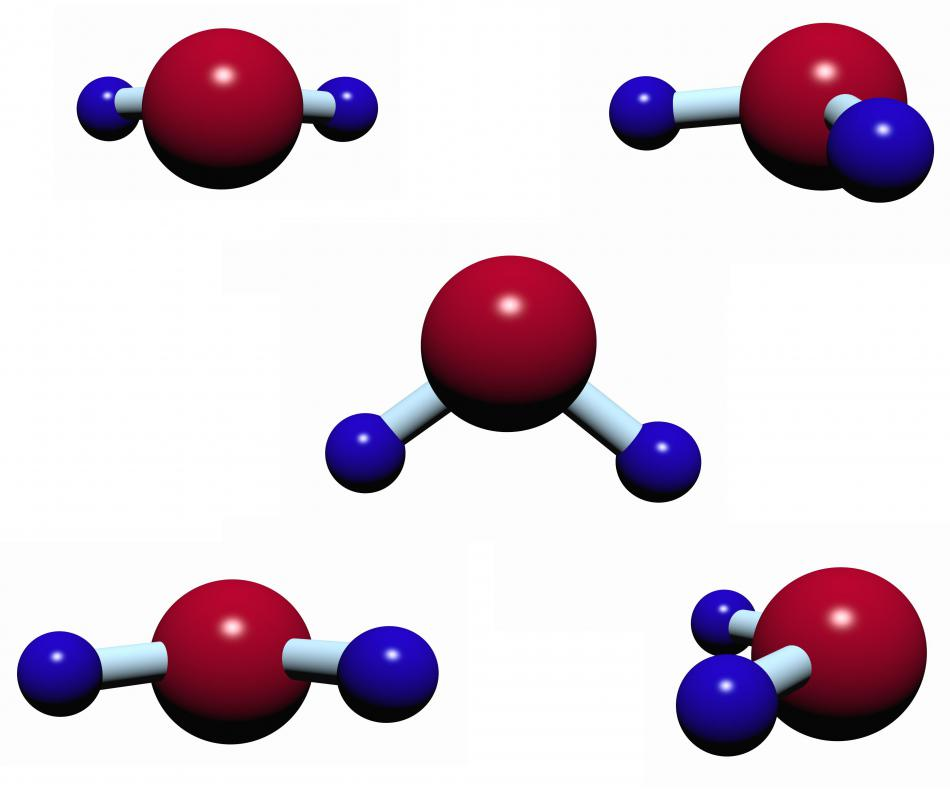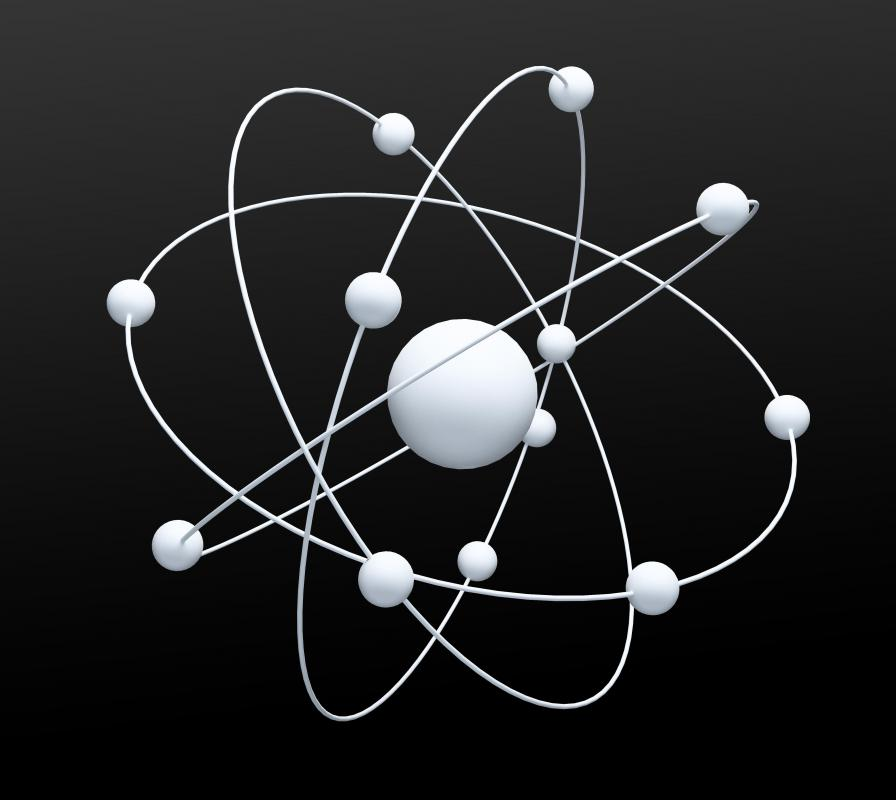# What Is Molar Mass? (with pictures)

Jillian O Keeffe
Jillian O KeeffeThe molar mass of water is equivalent to the mass of one mole of water molecules.

Molar mass is the mass of one mole of a substance. A mole is defined as the number of atoms in 12 grams of the carbon-12 isotope. This is always a fixed number, known as Avogadro's number. Therefore, the molar mass of a substance is the mass of Avogadro's number molecules of that substance. Molar mass is used in calculating concentrations of a substance in chemistry.Atoms often contain a proton, a neutron, and an electron.

Atoms contain at least two and usually three types of particles. These are the proton, the neutron, and the electron. A proton is roughly equivalent to the neutron in terms of mass. The electron is much lighter, so much as to be almost negligible when calculating mass.

One mole is a scientific form of measurement in chemistry. Elements need to have equal amounts of protons and electrons to be stable, but they can vary in the amount of neutrons they contain. The amount of protons in the element is the atomic number.Chemists use the mole as a form of measurement of mass and of concentration of a substance.

The amount of protons and neutrons together is the mass number because electrons are so light. Atomic number denotes the element. Mass number can change depending on the amount of neutrons the atom contains. Atoms that are the same element but have different masses are isotopes.

An atom of carbon always has six protons. If it has six neutrons as well, it is called the carbon-12 isotope. Made with eight neutrons, it is a carbon-14 isotope.

Scientists chose the carbon-12 isotope as the basis for mole measurements. Twelve grams of carbon-12 contains 6.022 x 1023 atoms. This is known as Avogadro's number. One mole of any substance is defined as this many atoms or molecules.

Cleverly, the mass of one mole of a substance relates to the individual mass number of the atoms in that substance. For example, one mole of oxygen has a mass of 16 grams. This is because the most common type of oxygen atom contains eight protons, eight neutrons, and eight electrons, and therefore, has a mass number of 16.

Measurement using molar mass is also possible with a compound molecule. Water is a molecule that has two hydrogen and one oxygen atoms stuck together. Adding all of their mass numbers together gives us the mass of one molecule of water. This number is equivalent to the mass in grams of one mole of water.

Chemists use the mole as a form of measurement of mass and of concentration of a substance. As a mole can contain trillions of atoms, and a given number of grams of one substance is not necessarily the same strength as the same number of grams of another substance, it is a very useful method of measuring chemicals relatively simply. The molar mass of a substance also equals the mass number of the substance, so chemists can easily use this known quantity to weigh out how much of the chemical they need to use.

## You might also Like## Discuss this Article

Forgot password?
###### Register:
•The molar mass of water is equivalent to the mass of one mole of water molecules.
•Atoms often contain a proton, a neutron, and an electron.
•Chemists use the mole as a form of measurement of mass and of concentration of a substance.